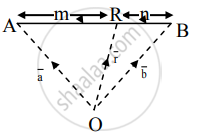# Let A(a¯) and B(b¯) are any two points in the space and R(r¯) be a point on the line segment AB dividing it internally in the ratio m : n, then prove that r¯=mb¯+na¯m+n - Mathematics and Statistics

Sum

Let "A" (bar"a") and "B" (bar"b") are any two points in the space and "R"(bar"r") be a point on the line segment AB dividing it internally in the ratio m : n, then prove that bar "r" = ("m"bar"b" + "n"bar"a")/("m" + "n")

#### Solution

R is a point on the line segment AB(A-R-B) and bar"AR" and bar"RB" are in the same direction.

Point R divides AB internally in the ratio m : n∴ "AR"/"RB" = "m"/"n"

∴ n(AR) = m(RB)

As n (bar"AR") and "m"(bar"RB") have same direction and magnitude,

"n"(bar"AR") = "m"(bar("RB"))

∴ "n"("OR" - bar"OA") = "m"(bar"OB" - bar"OR")

∴ "n"(bar"r" - bar"a") = "m"(bar"b" - bar"r")

∴ "n"bar"r" - "n"bar"a" = "m"bar"b" - "m"bar"r"

∴ "m"bar"r" + "n"bar"r" = "m"bar"b" + "n"bar"a"

∴ ("m" + "n")bar"r" = "m"bar"b" + "n"bar"a"

∴ bar"r" = ("m"bar"b" + "n"bar"a")/("m" + "n")

Concept: Section Formula
Is there an error in this question or solution?